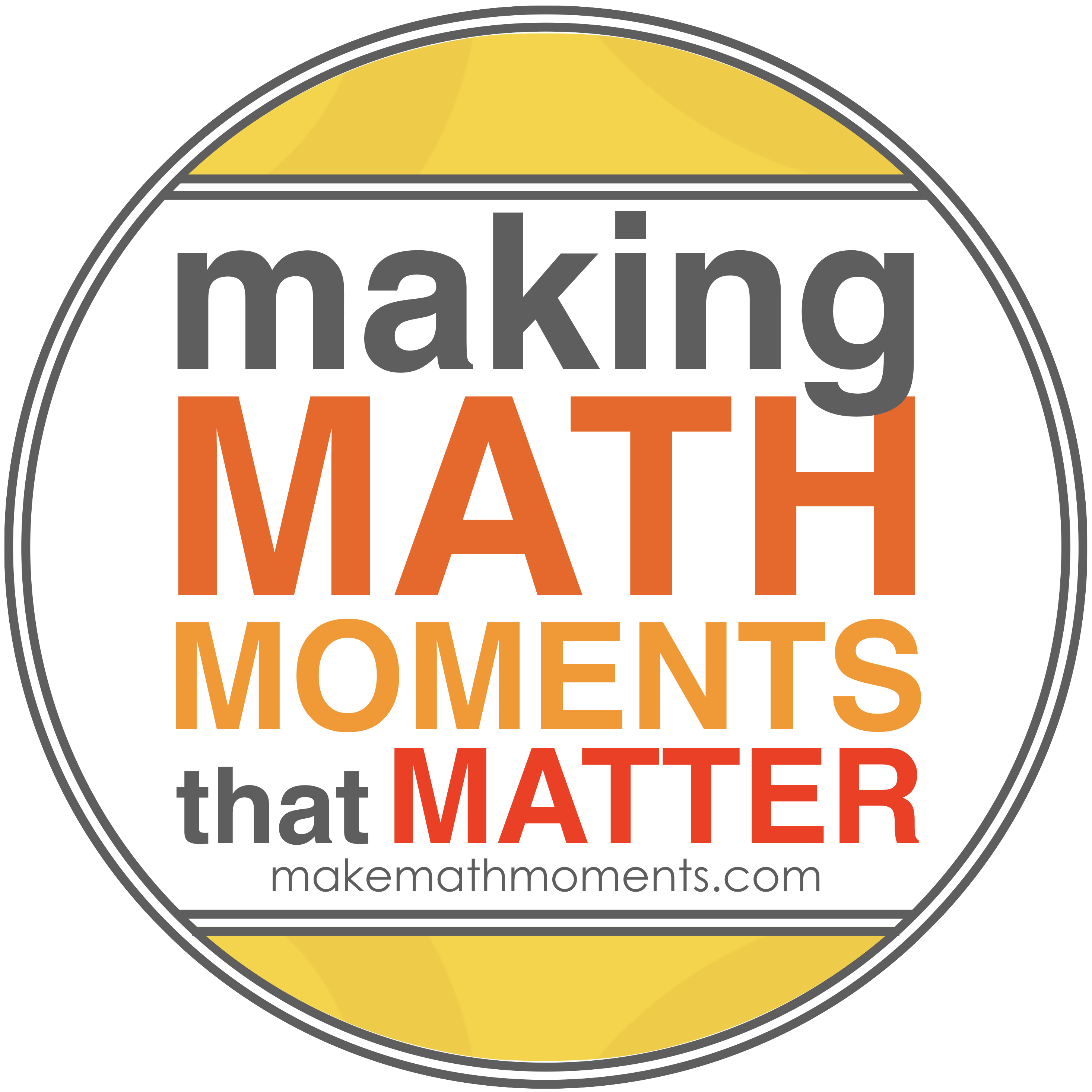# MBF3C

Course Description:

This course enables students to broaden their understanding of mathematics as a problem solving tool in the real world. Students will extend their understanding of quadratic relations; investigate situations involving exponential growth; solve problems involving compound interest; solve financial problems connected with vehicle ownership; develop their ability to reason by collecting, analysing, and evaluating data involving one variable; connect probability and statistics; and solve problems in geometry and trigonometry. Students will consolidate their mathematical skills as they solve problems and communicate their thinking.

Overall Learning Expectations:

By the end of this course, students will:

• make connections between the numeric, graphical, and algebraic representations of quadratic
• relations, and use the connections to solve problems;
• demonstrate an understanding of exponents, and make connections between the numeric, graphical, and algebraic representations of exponential relations;
• describe and represent exponential relations, and solve problems involving exponential relations arising from real-world applications;
• compare simple and compound interest, relate compound interest to exponential growth, and solve problems involving compound interest;
• compare services available from financial institutions, and solve problems involving the cost of making purchases on credit;
• interpret information about owning and operating a vehicle, and solve problems involving the associated costs;
• represent, in a variety of ways, two-dimensional shapes and three-dimensional figures arising from real-world applications, and solve design problems;
• solve problems involving trigonometry in acute triangles using the sine law and the cosine law, including problems arising from real-world applications;
• solve problems involving one-variable data by collecting, organizing, analysing, and evaluating data;
• determine and represent probability, and identify and interpret its applications.

-2-

Evaluation:

Term Work 70%

Knowledge / Understanding                                    35 %

Inquiry / Thinking                                                15 %

Communication                                                15 %

Application / Making Connections                        35 %

Assessment activities during the course will be comprised of a variety of methods and strategies.  (eg.  Assignments, projects, tests, journals, performances, conferences, daily work, etc.)

Final Evaluation 30%

The final evaluation will consist of, but is not limited to, a final examination, written during the examination schedule.

Classroom Rules:

Students are expected to follow these rules so that mathematics education can be successful.

a) BE ON TIME

b) COME PREPARED FOR CLASS

c) PARTICIPATE IN CLASS – Keep good notes while the lesson is being taught, pay

d) RESPECT THE RIGHTS OF OTHERS – Work quietly and co-operate so as not to

disturb other students who are trying to learn.

e) CATCH UP ON ANY WORK MISSED – When returning from an absence, catch up

on missed work as quickly as possible.  This is your responsibility.

f) COMPLETE ANY HOMEWORK ASSIGNED – If work is not completed in class,

complete it before the next class so that you may benefit from the discussions based on

the homework.

g) REMAIN IN YOUR DESK until the teacher dismisses the class.

h) FOLLOW ESTABLISHED SCHOOL ROUTINES AND BEHAVIOUR CODES – see

student handbook.

Learning Materials Required:

• Textbook
• Notebook – 3 ring binder with paper
• Pencil, eraser, ruler
• Scientific calculator
• Student Handbook## CONTACT ME